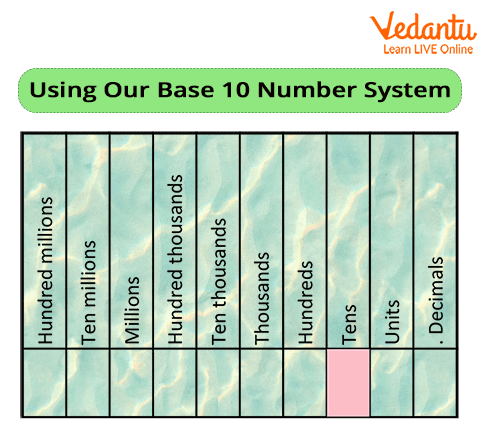Courses
Courses for Kids
Free study material
Free LIVE classes
More

# Base Ten Numerals## An Introduction to Base Ten Numerals in Maths

A number system allows us to describe a given number in bases. We know that 0 and 1 are used in binary form. However, what are base-ten numerals? Well, single-digit numbers from 1 to 9 are base-ten numerals. Also, we can only count to nine without the requirement for two numerals or digits. All numbers in the number system are formed by combining these 10 numerals or digits, especially when we talk about the decimal base in a number system.

So, let us begin our page with the base-10 or the decimal number system and apply this trick on various numbers to mentally exercise on finding out the base-10 of each.

## The Powers of 10

In base-10, each digit of a number is an integer value ranging from 0 to 9 (which means 10 possibilities) depending on its position. The place value or positions of the numbers are based on powers of 10. Each number position is 10 times the value to the right of it, thus the term base-10. Therefore, exceeding the number 9 in a position initiates counting in the next highest position.

• Ones

• Tens

• Hundreds

• Thousands

• Ten-thousands

• Hundred-thousands, etc.The example below will help you understand that values that are a fraction of or less than 1 in value appear to the right of the decimal point:

• Tenths

• Hundredths

• Thousandths

• Ten-thousandths

• Hundred-thousandths, and so on.

Every real number is expressible in base-10. Besides this, every rational number that has a denominator with only 2 and/or 5 as prime factors can be written as a decimal fraction. Therefore, these fractions have a finite decimal expansion.

On the other hand, all irrational numbers may be expressed as unique decimal numbers in which the sequence neither recurs nor ends, for example, π. Such leading zeros do not affect a number; however, trailing zeros may be significant in measurements.

## Using Base Ten Numerals

Let's look at an example of a large number and apply the concept of base-10 to determine each digit's place value or a position. For example, let’s consider the whole number 769,854. The position of each digit is as follows:

• 7 has a place value of 700,000.

• 6 has a value of 60,000.

• 9 has a value of 9,000.

• 8 has a value of 800.

• 5 has a value of 50.

• 4 has a value of 4.

Now, let us take the decimal number 479612.564.

• 4 has a place value of 400,000.

• 7 has a value of 70,000.

• 9 has a value of 9,000.

• 6 has a value of 600.

• 1 has a value of 10.

• 2 has a value of 2.

Please note that numbers greater than (more than) 1 appear to the left of a decimal point and have the following place values:

• 5 has a value of 5/10th.

• 6 has a value of 6/100th.

• 4 has a value of 4/1000th.

## Do You Know?

• Decimal fractions first came into use in China in the 1st century B.C.

• Did you know that the Yuki language of California uses base-8 (octal), counting the spaces between fingers rather than the digits?

Last updated date: 26th Sep 2023
Total views: 141.6k
Views today: 3.41k

## FAQs on Base Ten Numerals

1. What was the origin of the base 10 numeral system?

Base-10 is often used in modern civilizations and it was the most common system for ancient civilizations as well, most likely because humans have 10 fingers. Going back to 3000 B.C., Egyptian hieroglyphs display proof of a decimal system. Further, this system was handed over to Greece, although the Greeks and Romans used base-5 along with it.

However, other civilizations used a variety of bases. For example, the Mayans used base-20, probably from counting both hands and toes.

From the above text, we understand that base-10 numerals are very easy to understand and they date back to a time when various types of number systems were in use.

2. What is the practical use of binary and decimal or the base 10 numeral system?

Basic computing relies on a binary or base-2 number system in which there are only two digits: 0 and 1. We call 0 and 1 binary digits.

However, Computers also use base-10 to perform arithmetic operations like addition, subtraction, division, and multiplication.